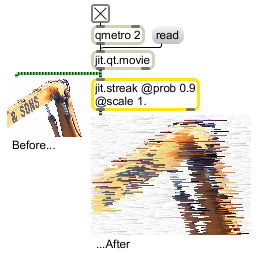# jit.streak

Probability lines

## Description

The jit.streak object uses a specified probability to determine the chance that a given matrix cell's value will be extended to subsequent cells (with an optional scaling factor). The result is a streaky good time.

## Matrix Operator

matrix inputs:1, matrix outputs:1
 Name IOProc Planelink Typelink Dimlink Plane Dim Type out n/a 1 1 1 1 1 char long float32 float64

## Attributes

Name Type g/s Description
direction int Streaking direction (default = 0 (left))
0 = left
1 = down
2 = right
3 = up
mode int Scanline wrapping mode (default = 0 (wrap))
0 = streaking wraps from scanline to scanline
1 = streaking begins anew at each scanline
prob float The probability that any given cell will be extended (streaked) (default = 0.)
scale float The scaling factor applied to extended (streaked) cells (default = 0.75)

## Examples